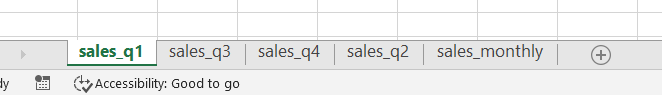Open in App
Not now

# How to get sheet names using openpyxl – Python

• Difficulty Level : Medium
• Last Updated : 09 Dec, 2022

The openpyxl library is widely used to handle excel files using Python. Often when dealing with excel files one might have noticed multiple sheets. The sheets are normally mentioned in the bottom left corner of the screen. One can install this library by running the following command.

`pip install openpyxl`

In this article, we will be seeing how to get the names of these sheets and display them using openpyxl.

The file we will be using here is named Example.xlsx and has the following sheets.## Python Codde to get sheet names using Openpyxl

First, we need to import the openpyxl library. After this, we will load our excel sheet Example.xlsx. Then by using the function sheetnames we can get a list of names of all the sub sheets that are present in the main sheet.

## Python3

 `# importing the openpyxl module as xl``import` `openpyxl as xl`` ` `# excel file used here gfg.xlsx``excel_file ``=` `"Example.xlsx"`` ` `# load the workbook``wb ``=` `xl.load_workbook(excel_file)`` ` `# print the list that stores the sheetnames``print``(wb.sheetnames)`` ` `# we can also get the name of the sheet which is active by using the active property``print``(``"Active sheet: "``, wb.active)`` ` `# By default the active sheet is the 1st one and can be changed``wb._active_sheet_index ``=` `4``sheet ``=` `wb.active``print``(``"Active sheet: "``, wb.active)`

Output:

```['sales_q1', 'sales_q3', 'sales_q4', 'sales_q2', 'sales_monthly']
Active sheet:  <Worksheet "sales_q1">
Active sheet:  <Worksheet "sales_monthly">```
My Personal Notes arrow_drop_up